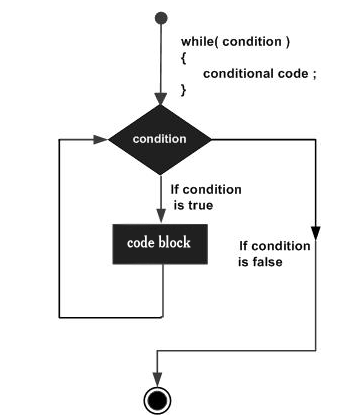×

Search anything:

# while loop in Java

#### Software Engineering while loop JavaGet this book -> Problems on Array: For Interviews and Competitive Programming

Reading time: 15 minutes | Coding time: 2 minutes

A while loop is an entry controlled control flow statement that allows code to be executed repeatedly based on a given condition. In Java, while loop is widely used.

Syntax

``````while (condition)
{
statement(s);
}
``````

Here, statement(s) may be a single statement or a block of statements.

The condition can be a logical or arithmatical combination of multiple small expressions which evaluate to true or any non-zero value. The loop iterates while the condition is true.

When the condition becomes false, program control passes to the instruction immediately after the loop.Note:-while loop might run forever which, will result as error in our source program.

Flow:

Step 1: Check condition
Step 2: If condition is True, go to Step 3. If condition is False, go to Step 5.
Step 3: Execute the code statements within while loop body
Step 4: Go to Step 1
Step 5: Exit while loop. Go to code statement immediately after while loop

When the condition is evaluated and the result is true, loop will be executed. We need to take care that after finite number of time our condition result into false in order to terminate execution.

## Example

Program to print all natural number less than or equal to '6' and greater than 0 using While loop.

``````class while_loop
{
public static void main (String[] args)
{
int i = 1;
while(i <= 6)
{
System.out.println(i);
++i;
}
}
}
``````

When the above code is compiled and executed, it produces the following result :-

``````1
2
3
4
5
``````

### Infinite loop using while loop

While loop can run infinitely if the condition always evaluates to true. Setting the condition to true will result in infinite loop.

Keeping the condition empty will give compilation error.

``````class while_loop
{
public static void main (String[] args)
{
while(true); // Infinite while loop

while(); // compilation error

while(1==1); // Infinite while loop
}
}
``````

### Benchmarking while loop vs for and do while loop

We will use the following code to perform this test:

``````class while_loop
{
public static void main (String[] args)
{
int count = 1;
long t1_1 = System.nanoTime();
while(count <= 1000000) count=count+1;
long t1_2 = System.nanoTime();

long t2_1 = System.nanoTime();
for(count=1; count <=1000000; count=count+1);
long t2_2 = System.nanoTime();

count = 1;
long t3_1 = System.nanoTime();
do{count=count+1;}while(count<=1000000);
long t3_2 = System.nanoTime();

System.out.println("Time taken by while loop: "+(t1_2-t1_1)+ " nanoseconds");
System.out.println("Time taken by for loop: "+(t2_2-t2_1)+ " nanoseconds");
System.out.println("Time taken by do while loop: "+(t3_2-t3_1)+ " nanoseconds");
}
}
``````

Output:

``````Time taken by while loop: 3602126 nanoseconds
Time taken by for loop: 1669825 nanoseconds
Time taken by do while loop: 7253640 nanoseconds
``````

Hence, we see, in terms of execution time while loop takes more time compared to for loop but takes nearly half the time taken by do while loop. Hence, while loop should be replaced by for loop whenever possible.

### do while > while > for

If execution time for for loop is taken as X, then:

for loop: X
while loop: 2X
do while loop: 4X#### OpenGenus Foundation

The official account of OpenGenus IQ backed by GitHub, DigitalOcean and Discourse

Improved & Reviewed by: